Get instant live expert help with Excel or Google Sheets“My Excelchat expert helped me in less than 20 minutes, saving me what would have been 5 hours of work!”

#### Post your problem and you'll get expert help in seconds

Your message must be at least 40 characters
Our professional experts are available now. Your privacy is guaranteed.

# Count specific words in a cell

While working with Excel, we are able to count specific words or text by using the LEN and SUBSTITUTE function.  LEN returns the length of a text string while SUBSTITUTE replaces a word in a text string.  This step by step tutorial will assist all levels of Excel users in counting specific words in a cell.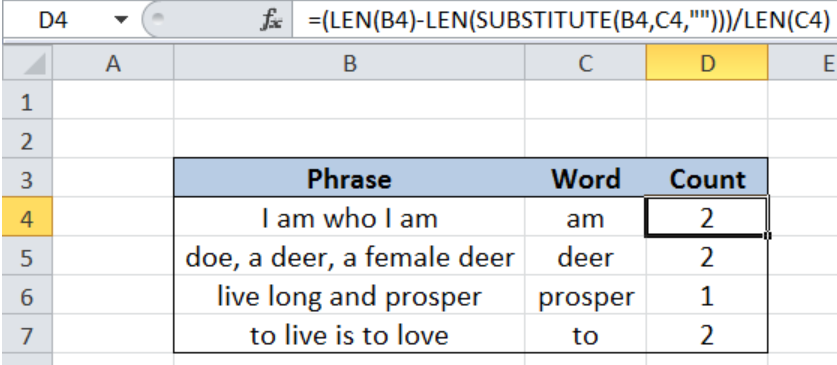Figure 1. Final result: Count specific words in a cell

Final formula:  `=(LEN(B4)-LEN(SUBSTITUTE(B4,C4,"")))/LEN(C4)`

## Syntax of the LEN function

LEN returns the length of a text string in number of characters

`=LEN(text)`

• text  – the text whose length we want to determine. Spaces are counted as characters.

## Syntax of the SUBSTITUTE function

SUBSTITUTE function replaces a word or text in a text string

`=SUBSTITUTE(text, old_text, new_text, [instance_num])`

• text  – the text string containing the word or text we want to replace or change
• old_text  – any text that we want to replace with new_text
• new_text  – the text we want to replace old_text with
• instance_num  – Optional; Specifies which occurrence of old_text we want to replace; If omitted, every occurrence of old_text in text is replaced with new_text

## Setting up Our Data

Our table contains three columns: Phrase (column B), Word (column C) and Count (column D).  We want to know how many times the word in column C occurs in column B. The resulting count will be recorded in column D.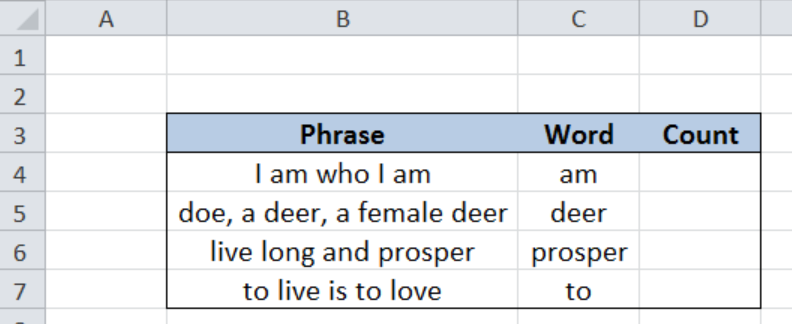Figure 2. Sample data to count specific words in a cell

## Count specific words

In order to count specific words occurring in a cell, we follow these steps:

Step 1.  Select cell D4

Step 2.  Enter the formula: `=(``LEN(B4)-LEN(SUBSTITUTE(B4,C4,"")))/LEN(C4)`

Step 3.  Press ENTER

Step 4.  Copy the formula in cell D4 to cells D5:D7 by clicking the “+” icon at the bottom-right corner of cell D4 and dragging it down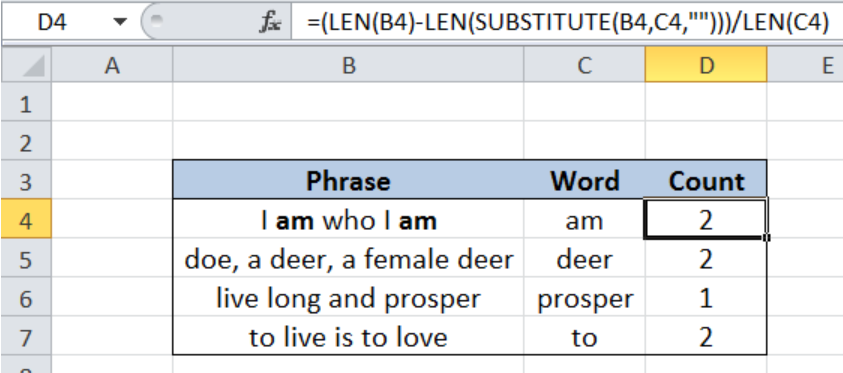Figure 3. Count specific words using LEN and SUBSTITUTE

SUBSTITUTE replaces the word “am” in B4 with an empty string “”.  The first LEN function returns the length of our phrase in B4, while the second LEN returns the length of the resulting phrase in B4 without the word “am”.  The result is then divided by the length of the word “am” in C4.

The final result in cell D4 is 2, which is the number of times that the word “am” occurs in the phrase “I am who I am”.

## Work-around to case sensitivity

The SUBSTITUTE function is case sensitive.  Let us consider below example where the first letter is a capital letter.  Entering our formula in cell D4 will return the value 1. The formula counts only the second occurrence of the word “to”.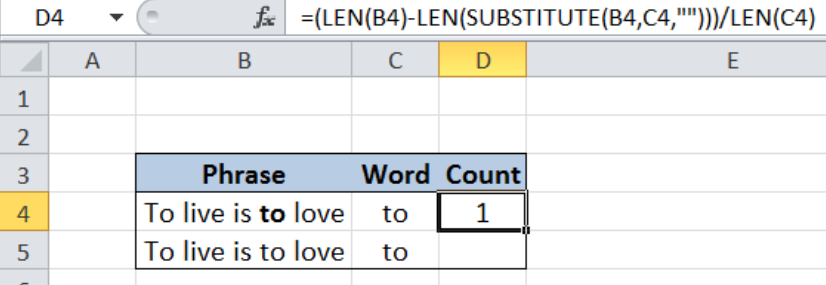Figure 4. Incorrect count of the word “to” due to case sensitivity of SUBSTITUTE

The work-around for the case-sensitivity of the SUBSTITUTE function is by using the UPPER function.

## Syntax of the UPPER function

UPPER function converts any text to uppercase

`=UPPER(text)`

• text – The text we want to convert to uppercase; can be a reference or text string

Step 1.  Select cell D5

Step 2.  Enter the formula: `=(LEN(B5)-LEN(``SUBSTITUTE(UPPER(B5),UPPER(C5),"")))/LEN(C5)`

Step 3.  Press ENTER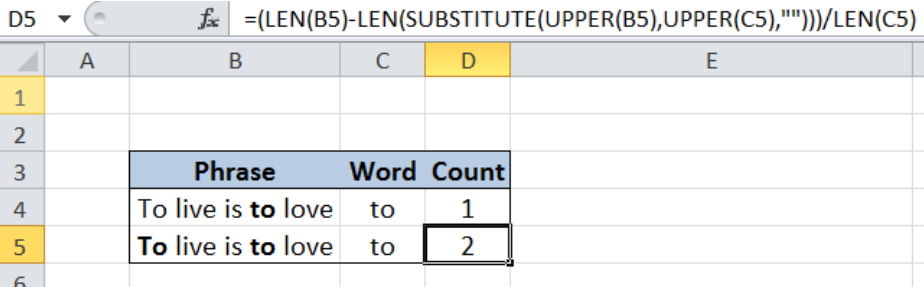Figure 5. Output: Correct count by using UPPER with LEN and SUBSTITUTE

The UPPER function inside the SUBSTITUTE function converts both the phrase in B5 and the word in C5 to uppercase before substitution takes place.  As a result, the formula correctly counts that the word “to” occurs twice in the phrase “To live is to love.”

Most of the time, the problem you will need to solve will be more complex than a simple application of a formula or function. If you want to save hours of research and frustration, try our live Excelchat service! Our Excel Experts are available 24/7 to answer any Excel question you may have. We guarantee a connection within 30 seconds and a customized solution within 20 minutes.

### Did this post not answer your question? Get a solution from connecting with the expert.Another blog reader asked this question today on Excelchat:## Subscribe to Excelchat.coAnother blog reader asked this question today on Excelchat: